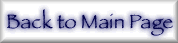## 2. Form Factors and Standard Definitions

The (x-ray) atomic form factor f is the resonant scattering amplitude of x-rays by charge (primarily electron) density. Using standard conventions in the x-ray regime, we also consider the imaginary and real components of the form factor separately, and separate three contributions to the real component. The real component Re(f) is composed of: the "normal" coherent scattering factor f0, depending upon the photon angle of scattering θ via the momentum transfer(eq 3)

with λ in, e.g., Ångstroms; the "anomalous" scattering factor f′ (depending on x-ray energy E and the atomic number Z); and the small nuclear Thomson term fNT [10,11]. f′ can also be expressed in terms of a small relativistic correction term frel, Z and the function f1 often used to characterize these form factors:(eq 4)(eq 5)

The angular factor f0 is identical to the values f(q) or F(x,Z) given in Hubbell et al. , Hubbell and Øverbø , and Schaupp et al.  and use q instead of x, with x = q/4π.(eq 6)

The imaginary component Im(f) = f″ is directly related to the atomic photoabsorption cross-section given as τPE or σPE in different references:(eq 7)

Fundamental constants and conversion factors are given in ref. . Conventionally, the total interaction cross-section σtot is represented as a sum over the individual photon interaction cross-sections:(eq 8)

These cross-sections would conventionally be given in barns/atom. This would be directly related to the linear attenuation coefficient (µ) in cm-1, and the mass attenuation coefficient in cm2/g. The mass attenuation coefficient is conventionally given by the symbol [µ/ρ] = σ/uA, where σ is the cross-section in barns/atom, u is the atomic mass unit, and A is the relative atomic mass of the target element. Coefficients for converting between these units are given by many authors . (See the table header in section 13 and the element header information accessed through the online database.)

This paper develops the approach covered in Chantler  and makes extensive reference to this earlier work, which will therefore be denoted in what follows as C95. Table 1 summarizes the type of use to which this tabulation (and that of C95) may be put. It summarizes the typical equation to use (with reference to column headings in the current tabulation) and gives the author's current personal recommendation of a useful or appropriate reference for additional information or coefficients as might be needed.

 Form factors for forwarding scattering section 8.1 Direct use or interpolation, with (eq 4) Form factors for significant momentum transfers section 8.2 (eq 4) and (eq 5) or refs [13,14],  or  Calculation of structure factors section 8.3 As section 8.2 but also (eq 1) Refractive indices section 8.3 As section 8.2 but also (eq 2) Crystallography (diffraction) section 8.4 As section 8.3 but also see text for references Multilayer reflectivity, transmission section 8.4 As section 8.2 and section 8.3 but also see text for references Electron density studies section 8.5 As section 8.3 but also (eq 11) Sum rules section 8.6 As section 8.2 but also see text for references Computation of scattering processes section 8.7 As section 8.2 but also (eq 12), (eq 14), (eq 1), (eq 16), (eq 17), (eq 18), and (limited) (eq 13) and (eq 15) (see text) Photoelectric cross-sections, linear absorption coefficient, or mass absorption coefficients section 8.8 Direct use or interpolation, with conversion as given in table headers as needed X-ray Attenuation [Medical imaging, transmission studies] section 8.8 Direct use of total mass attenuation coefficient for Raleigh scattering X-ray Attenuation studies with alternate scattering estimates section 8.8 Direct use of mass absorption coefficient, with (eq 19) and possibly refs. , ,  X-ray Attenuation of crystalline samples section 8.8 Direct use of mass absorption coefficient, with (eq 19) and section 8.7 Angle-dependent scattering processes section 8.8 Not applicable in general - see text High-energy attenuation, above 100 keV section 8.9 Direct use of mass absorption coefficient, with (eq 19), (eq 8), and possibly refs , [12,13], and  High-energy (γ-ray) attenuation, above 1 MeV section 8.9 See refs [12,13] VUV studies section 8.10 Directly, but with caveats and see also ref.  K-shell studies & fluorescence yields section 8.11 Directly, but see text Electron scattering section 8.14 (eq 21), and see text